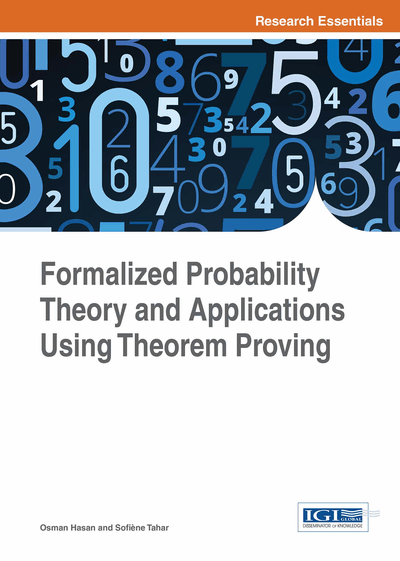# Applications of Formalized Information Theory

DOI: 10.4018/978-1-4666-8315-0.ch011

## Abstract

In previous chapters, the authors provided a comprehensive framework that can be used in the formal probabilistic and information-theoretic analysis of a wide range of systems and protocols. In this chapter, they illustrate the usefulness of conducting this analysis using theorem proving by tackling a number of applications including a data compression application, the formal analysis of an anonymity-based MIX channel, and the properties of the onetime pad encryption system.
Chapter Preview
Top

## 11.1 Data Compression

Data compression or source coding may be viewed as a branch of information theory (Cover & Thomas, 1991) in which the primary objective is to reduce redundancy, minimizing the amount of data to be stored or transmitted. It consists in encoding information using fewer bits than an un-encoded representation would use, through use of specific encoding schemes. As depicted in Figure 1, data compression has important applications in the areas of data storage and data transmission, for instance, in the speech compression for real time transmission over digital cellular networks. On the other hand, the proliferation of computer communication networks is resulting in massive transfer of data and the growing number of data processing applications require storage of large volumes of data. Compressing data reduces costs and increases capacity. However, to guarantee reliable transmission or storage of data, particularly in systems for safety-critical applications, a certain limit of compression must be respected.

We propose to formally prove an important result establishing the fundamental limit of data compression. This result is also known as the Shannon source coding theorem (Affeldt & Hagiwara, 2012) and states that it is possible to compress the data at a rate that is arbitrarily close to the Shannon entropy without significant loss of information (Gray, 1990). In other words, n independent and identically distributed (iid) random variables with entropy H (X) can be expressed on the average by n H (X) bits without significant risk of information loss, as n tends to infinity. To the best of our knowledge, most of the formalization framework that we need for this application, such as the properties of real valued measurable functions, properties of the expectation of arbitrary functions, variance, independence of random variables and the weak law of large numbers, is not available in the open literature.

A proof of this result consists in proposing an encoding scheme for which the average code word length can be made arbitrarily close to n H (X) with negligible probability of loss. We propose to perform a typical set encoding. The typical set contains the typical sequences, which are used to represent the frequent source symbols while the non-typical sequences represent rare source symbols.

The encoding is performed separately for the typical and the non-typical set as depicted in Figure 2. Clearly, we need to formalize the concept of a typical set and prove its properties to be able to formally verify that the average code word length associated to the typical-set encoding can be made arbitrarily close to the Shannon entropy with a vanishing probability of error. To prove the properties of the typical set, we need to prove the Asymptotic Equipartition Property (AEP), which in turn, requires the proof of the classical probability results, namely, the Weak Law of Large Numbers (WLLN) and the Chebyshev inequality.

## Complete Chapter List

Search this Book:
Reset